# Current Mirror Circuit: Wilson and Widlar Current Mirroring Techniques

Published  June 4, 2019   0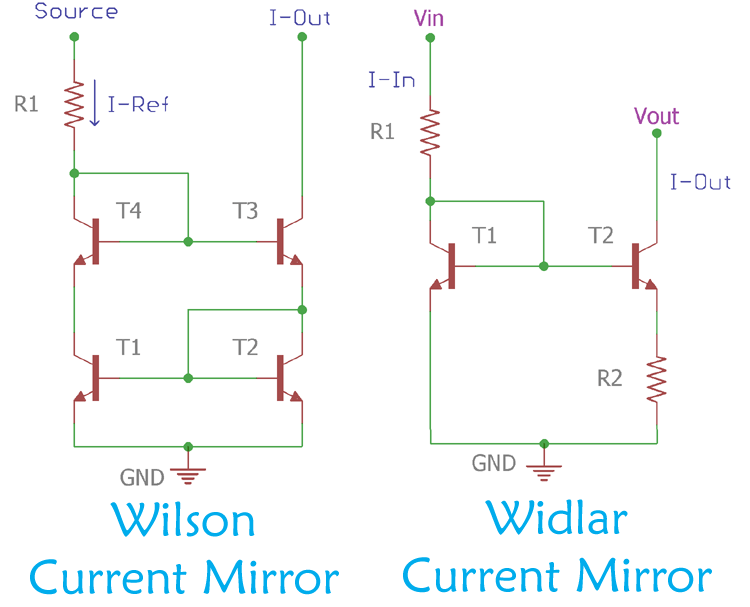In the previous article, we discussed about Current Mirror Circuit and how it can be built using Transistor and MOSFET. Despite the fact that basic current mirror circuit can be constructed using two simple active components, BJTs and MOSFETs or using an amplifier circuit, the output is not perfect, as well as it has certain limitations and dependencies on the external things. So to get a stable output, additional techniques are used in current mirror circuits.

### Improving the Basic Current Mirror Circuit

There are several options to improve the output of Current Mirror Circuit. In one of the solution one or two transistors are added over the traditional two transistors design. Construction of those circuits uses emitter follower configuration to overcome the base current mismatch of the transistors. The design can have a different kind of circuit structure to balance the output impedance.

There are three primary metrics to analyze the current mirror performance as part of a large circuit.

1. First metric is the amount of static error. It is the difference between input and output currents. It is a tough task to minimize the difference as the difference of differential single-ended output conversion with the differential amplifier gain is responsible to control the rejection ratio of common mode and power supply.

2. The next most crucial metric is the current source output impedance or the output conductance. It is crucial because it affects the stage again during the current source is acting like an active load. It also affects the common mode gain in different situations.

3. For the stable operation of current mirror circuits, the last important metric is the minimum voltages coming from the power rail connection located across the input and output terminals.

So to improve the output of Basic Current Mirror Circuit, considering all the above performance metrics, here we will discuss to popular Current Mirror Techniques - Wilson Current Mirror Circuit and Widlar Current Source Circuit.

### Wilson Current Mirror Circuit

It's all started with a challenge between two engineers, George R. Wilson and Barrie Gilbert, to make an improved current mirror circuit overnight. Needless to say that George R. Wilson won the challenge in 1967. From the name of George R. Wilson, the improved current mirror circuit designed by him is called Wilson Current Mirror Circuit.

Wilson current mirror circuit uses three active devices that accept the current across its input and provide the exact copy or mirrored copy of the current to its output.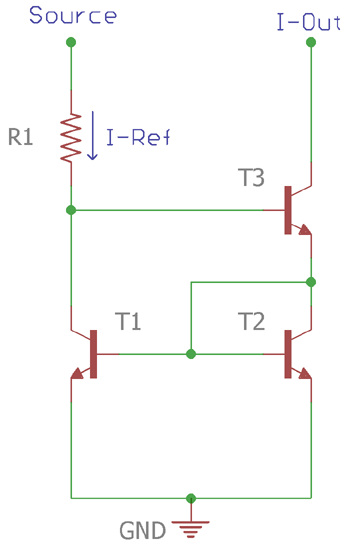In above Wilson Current Mirror Circuit, there are three active components which are BJTs and a single resistor R1.

Two assumptions are made here – one is that all transistors have the same current gain which is and second is that collector currents of T1 and T2 is equal, as the T1 and T2 are matched and the same transistor. Therefore

`IC1 = IC2 = IC`

And this applies for the base current too,

`IB1 = IB2 = IB`

The base current of the T3 transistor can easily be calculated by the current gain, which is

`IB3 = IC3 / β … (1)`

And the emitter current of the T3 will be

`IB3 = ((β + 1)/ β) IC3 … (2)`

If we look at the above schematic, the current across the T3 emitter is the sum of T2’s collector current and base currents of T1 & T2. Therefore,

`IE3 = IC2 + IB1 + IB2`

Now, as discussed above, this can be further evaluated as

```IE3 = IC + IB + IB
IE3 = IC + 2IB```

Hence,

`IE3 = (1+(2/β)) IC`

The IE3 can be changed as per the (2)

`((β+1)/ β)) IC3 = (1+(2/β)) IC`

The collector current can be written as,

`IC = ((1+ β) / (β + 2)) IC3 … (3)`

Again as per the schematic the current through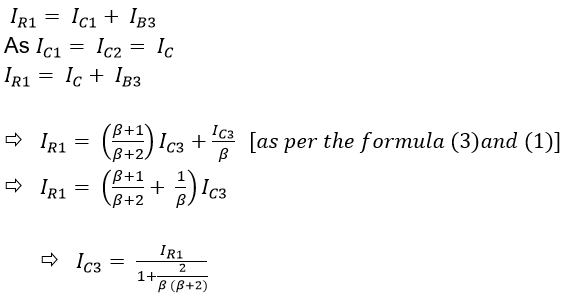The above equation can draw a relationship between the Third transistor collectors current with the input resistor. How? If 2/ (β(β + 2)) << 1 then the IC3 ≈ IR1. The output current can also be easily calculated if the base-emitter voltage of the transistors is less than 1V.

`IC3 ≈ IR1 = (V1 – VBE2 – VBE3) / R1`

So, for the proper and stable output current, the R1 and V1 need to be in proper values. To make the circuit act as a constant current source, the R1 need to be replaced with a constant current source.

Improving the Wilson Current Mirror Circuit

Wilson current mirror circuit can be further improved to get perfect accuracy by adding another transistor.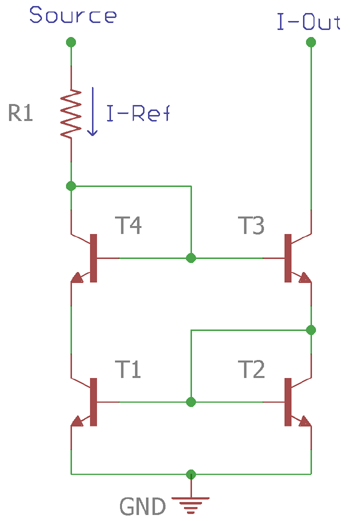The above circuit is the improved version of the Wilson current mirror circuit. The fourth transistor T4 is added in the circuit. The additional transistor T4 balances the collector voltage of T1 and T2. The collector voltage of T1 is stabilized by the amount equal to the VBE4. This results in finiteand also stabilize the voltage differences between the T1 and T2.

### Advantages and Limitation of Wilson Current Mirror Technique

The current mirror circuit has several advantages in comparison with traditional basic Current Mirror circuit-

1. In case of basic current mirror circuit, the base current mismatch is a common problem. However, this Wilson current mirror circuit virtually eliminates the base current balance error. Due to this, the output current is near to accurate as of the input current. Not only this, the circuit employs very high output impedance due to the negative feedback across the T1 from the base of the T3.
2. The improved Wilson current mirror circuit is made using 4 transistor versions so it is useful for the operation at high currents.
3. The Wilson current mirror circuit provides low impedance at the input.
4. It doesn't require additional bias voltage and minimum resources are needed to construct it.

Limitations of Wilson Current Mirror:

1. When the Wilson current mirror circuit is biased with maximum high frequency the negative feedback loop cause instability in frequency response.
2. It has a higher compliance voltage compared with the basic two transistor current mirror circuit.
3. Wilson current mirror circuit creates noise across the output. This is due to the feedback which raises output impedance and directly affect the collector current. The collector current fluctuation contributes noises across the output.

### Practical Example of Wilson Current Mirror Circuit

Here the Wilson current mirror is simulated using Proteus.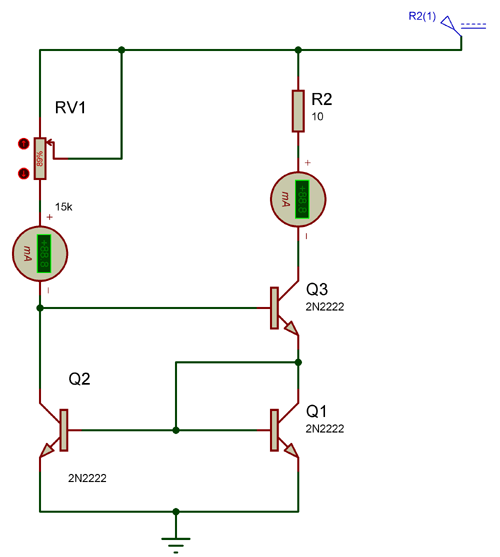The three active components (BJT) are used to make the circuitry. The BJTs are all 2N2222, with same specifications. The pot is selected to change the current across Q2 collector which will further reflect on the Q3 collector. For the output load, a 10 Ohms resistor is being selected.

Here is the simulation video for Wilson Current Mirror Technique-

In the video, the programmed voltage across Q2’s collector is reflecting across the Q3 collector.

### Widlar Current Mirror Technique

Another excellent current mirror circuit is the Widlar Current Source Circuit, invented by Bob Widlar.

The circuit is exactly the same as the basic current mirror circuit using two BJT transistors. But there is a modification in the output transistor. The output transistor uses an emitter degeneration resistor to provide low currents across the output using only moderate resistor values.

One of the popular application examples of Widlar current source is in the uA741 operational amplifier circuit.

In the below image, a Widlar current source circuit is shown.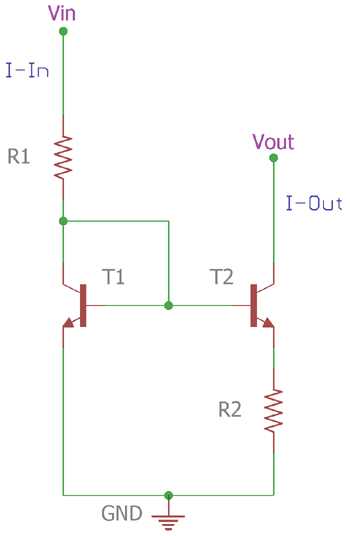The circuit consists of only two transistors T1 & T2 and two resistors R1 & R2. The circuit is same as the two transistors current mirror circuit without the R2. The R2 is connected in series with the T2 emitter and ground. This emitter resistor effectively reduces the current across the T2 compared with the T1. This is done by the voltage drop across this resistor, this voltage drop reduces the base-emitter voltage of the output transistor which further results in reduced collector current across the T2.

### Analyzing and deriving Output Impedance for Widlar Current Mirror Circuit

As previously mentioned that the current across T2 is reduced in comparison with T1 current, that can be further tested and analyzed using Cadence Pspice simulations. Let’s see the Widlar circuit construction and simulations in the below image,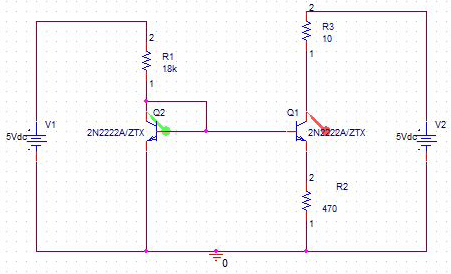The circuit is constructed in Cadence Pspice. Two transistors with the same specification are used in the circuitry, which is 2N2222. The current probes are showing the current plot across Q2 and Q1 collector.

The simulation can be seen in the below image.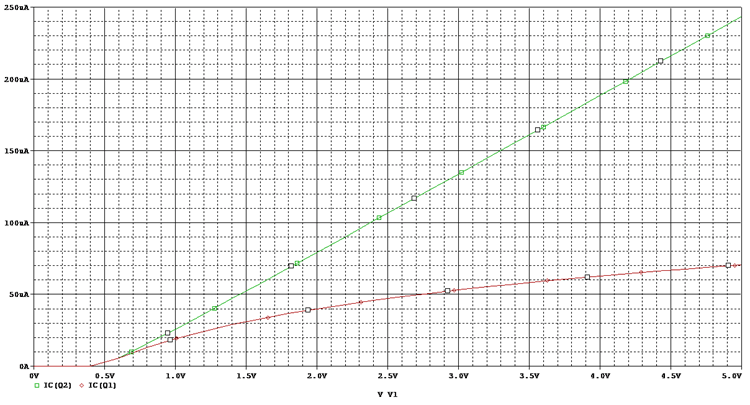In the above figure, the red plot, which is the collector current of Q1 is reducing while compared with Q2.

Applying KVL (Kirchhoff’s Voltage Law) across the base-emitter junction of the circuit,

```VBE1 = VBE2 + IE2R2
VBE1 = VBE2 + (β+1)IB2R2```

The β2 is for the output transistor. It is completely different from the Input transistor as the current plot on the simulation graph clearly shows that the current in two transistors are different.

The final formula can be drawn from the above formula if the finite β is overruled and if we change the IC1 as IIN and IC2 as IOUT. Therefore,

To measure the output resistance of the Widlar current source, the small-signal circuit is a useful option. The below image is an equivalent small signal circuit for Widlar current source.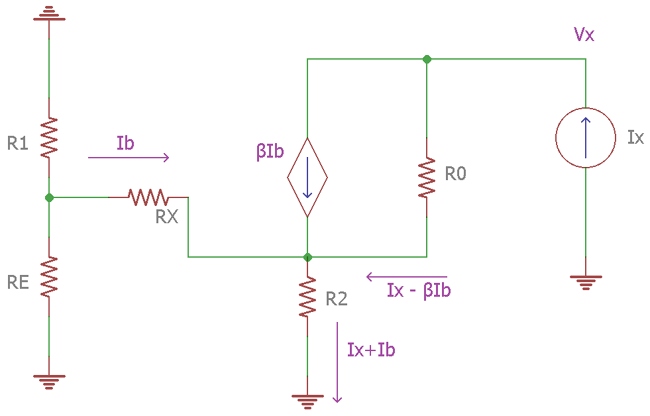The current Ix is applied across the circuit to measure the output resistance of the circuit. So, as per the Ohms law, the output resistance is

`Vx / Ix`

The output resistance can be determined by applying Kirchoff's law across the left ground to the R2, it is-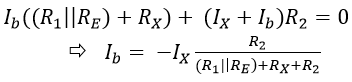Again, applying Kirchhoff’s voltage law across the R2 ground to the ground of Input current,

`VX = IX (R0 + R2) + Ib (R2 – βR0)`

Now, changing the value, final equation to derive the output resistance of Widlar Current Mirror circuit is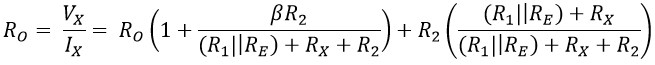So this is how the Wilson and Widlar current Mirror Techniques can be used to improve the designs of Basic Current Mirror Circuit.